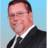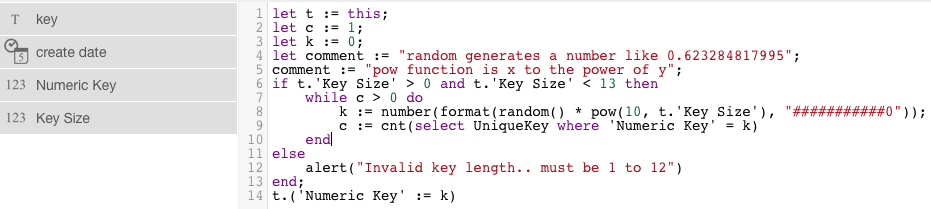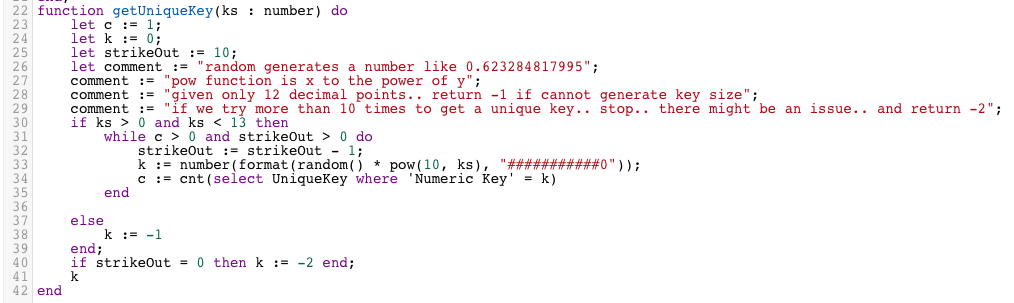Generate Unique Numeric Key for tableLets assume you want to create your one unique numeric key for a table object and you want to ensure it is of a fixed number of digits..

Here is a quick hack..   (put this code in a global function)

Table Name : UniqueKey (your table might be Invoice, Customer, Project, etc... )
Field 'Key Size' must be a numeric value between 1 and 12 (inclusive).. of course you can hard code it for your needs..

The code..If you have other approaches.. would like to see them..OK Folks.. here is the code as a global function... WHY a global function you may ask????   Because when you execute something like..

let uk := create UniqueKey;

Ninox will NOT execute the "on create trigger" .. therefor .. I put it into a global function so I can call it after I create a row via a script.PS.. I have an email into support.. For some reason.. I am getting a strange error that if this is the FIRST (or only) global function .. AND I was passing in number from a form field.... it would pass the number in as empty string.. Not sure why..

Can you post the raw formula to this please, Mconneen?

Thank you,

S Moore@SMoore,

Sure.... I usually post them as "images" because they format better.. :)

`function getUniqueKey(ks : number) do`
`let c := 1;`
`let k := 0;`
`let strikeOut := 10;`
`let comment := "random generates a number like 0.623284817995";`
`comment := "pow function is x to the power of y";`
`comment := "given only 12 decimal points.. return -1 if cannot generate key size";`
`comment := "if we try more than 10 times to get a unique key.. stop.. there might be an issue.. and return -2";`
`if ks > 0 and ks < 13 then`
`while c > 0 and strikeOut > 0 do `
`strikeOut := strikeOut - 1;`
`  k := number(format(random() * pow(10, ks), "###########0"));`
`  c := cnt(select UniqueKey where 'Numeric Key' = k)`
`end`

`else`
`   k := -1`
`end;`
`if strikeOut = 0 then k := -2 end;`
`k`
`end`